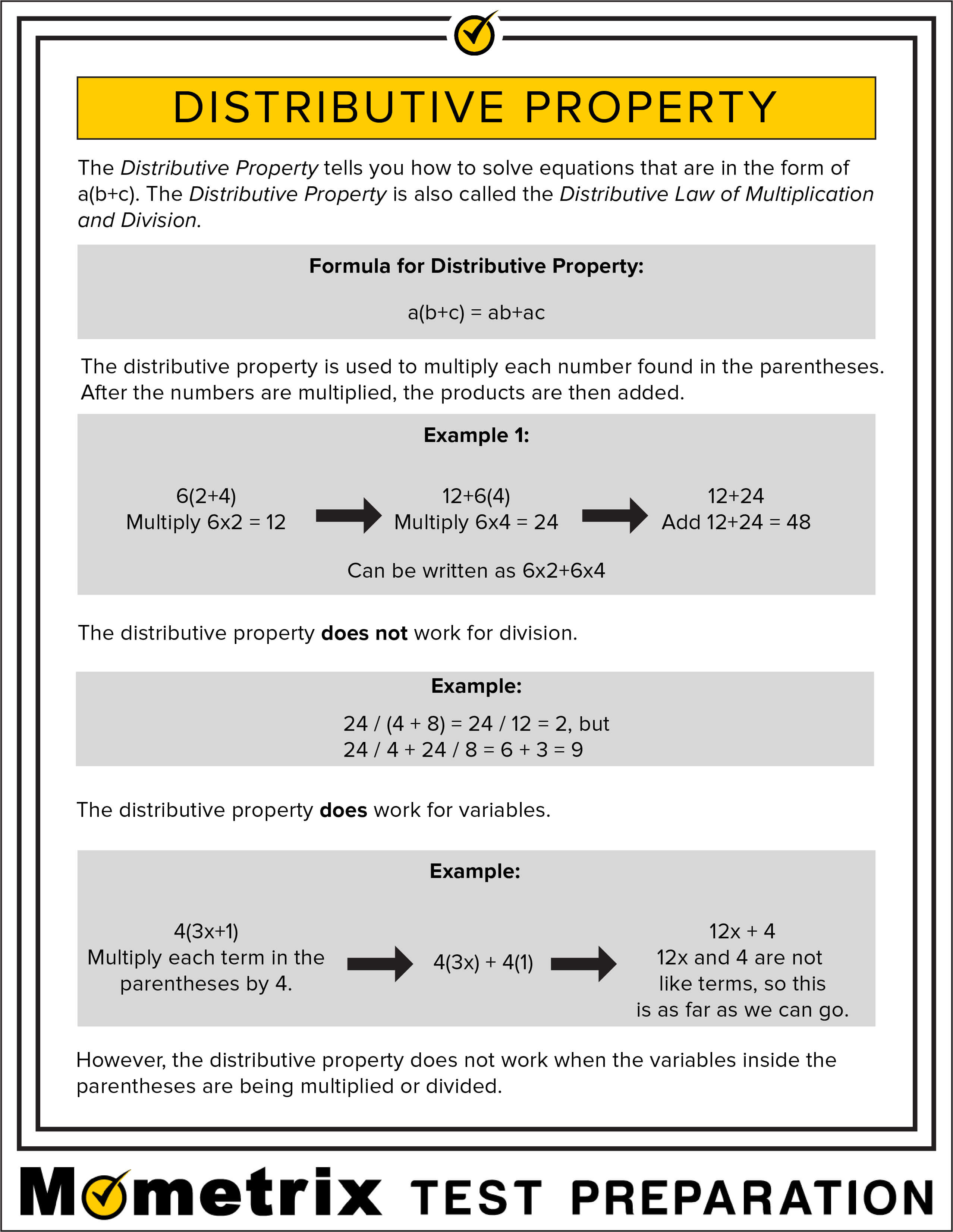# What is Distributive Property?

The distributive property involves multiplying over grouped addition or subtraction terms. It states that you multiply the number outside the parentheses by each term inside the parentheses and then add or subtract as in the original.

## Distributive Property

I want to briefly revisit a concept that I glossed over earlier. That’s the distributive property. The distributive property applies to all real numbers. Any time you are dealing with real numbers you can use the distributive property. The distributive property says that for any three real numbers (A, B, and C) that A plus B times C is equal to A times C plus B times C.

It’s called the distributive property, because you’re distributing this number here outside of the sum to each of the terms in the sum. This C is being distributed to A and to B. Because this distributed property works, you have a lot of different tools you can use to simplify expressions. I’m going to demonstrate a couple of those with a polynomial expression.

Let’s take the polynomial expression x to the third plus 3x squared plus 3x to the third plus 5x squared plus 6x. We have this long expression here and we want to simplify it to make it easier to solve an equation that has this in it. To do that, we’re going to apply the distributive property a couple of different ways. First, we’re going to use this to combine like terms.

You’ll notice we have this x cubed here plus 3x cubed. What we can do here is we can do this. Of course, it’s assumed to have a 1 before it. Essentially, we have 1 times x cubed plus 3 times x cubed. You’ll notice that’s very similar to what we have up here. We know that we can rewrite that expression in this format here, or as 1 plus 3, taking the A and B from over here and putting them in parentheses, times C, or x cubed.

This is the same as 4x cubed. Similarly, with the x squared terms, we can take the 3x squared plus the 5x squared and once again move from this format to this format. That will give us 3 plus 5 times x squared, or 8x squared. Now we can rewrite this expression here as 4x cubed plus 8x squared plus 6x. Now that we’ve combined like terms, we just have one term for each power of x, but we can take this one step further yet.

Now that we’ve combined like terms, we can pull out some terms that are common to each of these three terms. If you’ll notice, all three of these coefficients are even, which means that they’re all divisible by 2. We can pull out a 2. They all contain a power an x, so we can pull out an x. Let’s divide each of these terms by 2x and put that outside of the set of parentheses. 4x cubed divided by 2x is going to be 2x squared.

Plus we had 8x squared here, so divided by 2x is going to be plus 4x. Then 6x divided by 2x is just going to be three. We’ll multiply that now by 2x, because we pulled the 2x out of each term. Applying the distributive property here to this expression, we were able to simplify it to that form. There.

## Free Distributive Property Fact Sheet

Use the distributive property fact sheet below to help you get a better understanding of how distributive property work. You are encouraged to print or download the distributive property fact sheet with the PDF link at the bottom of the page.